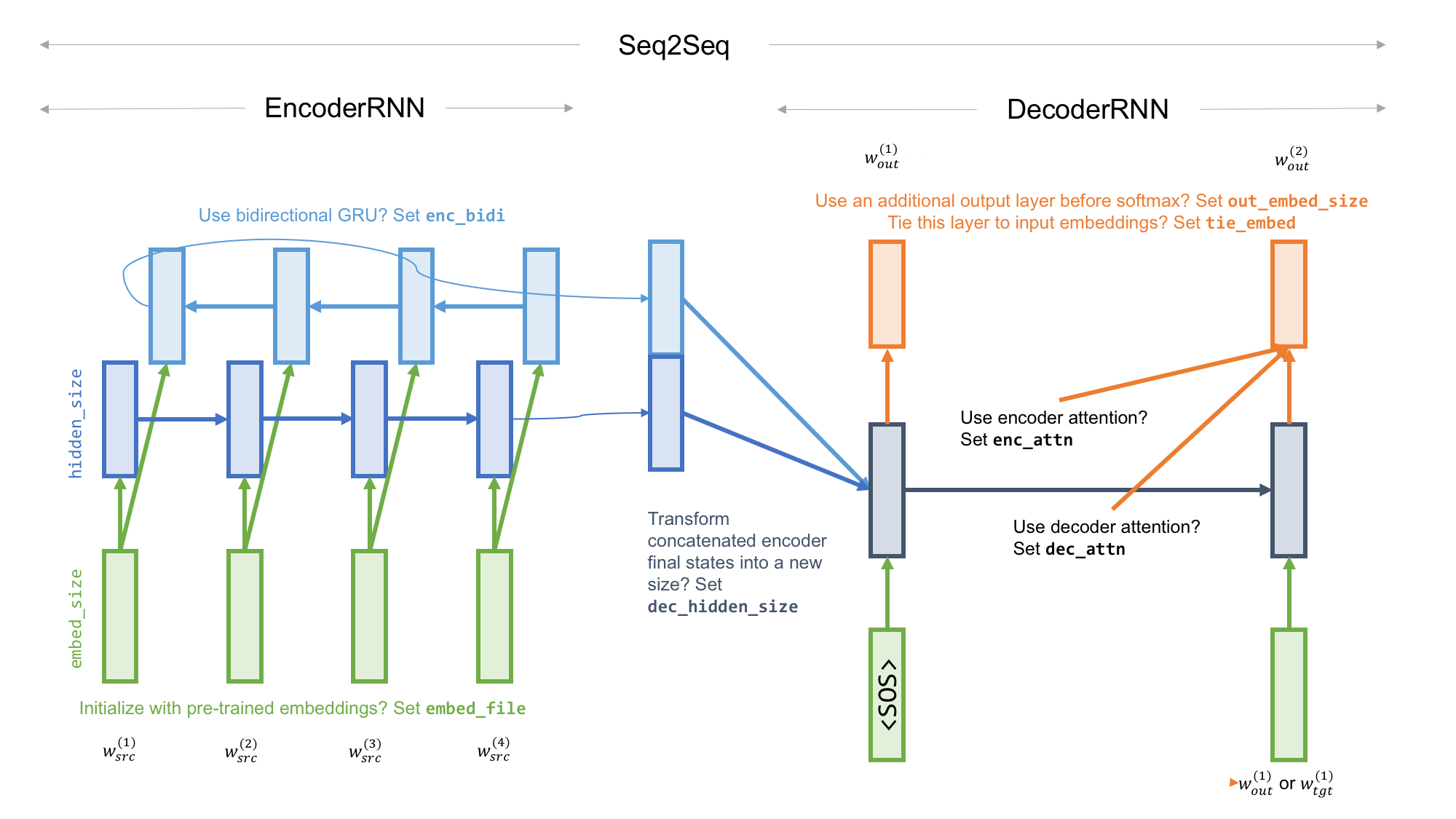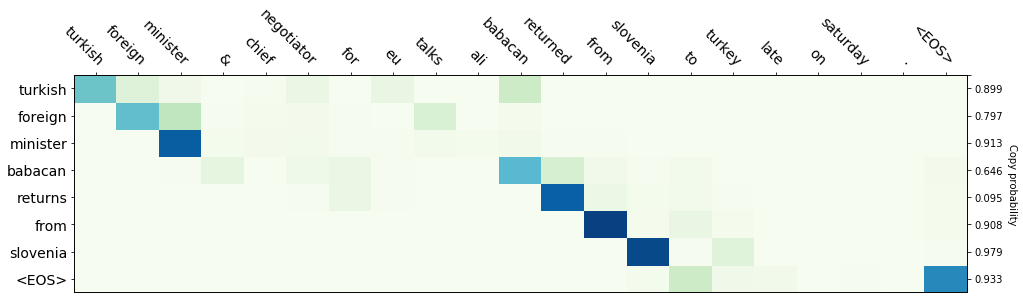## Quick start

#### Installation

The summarizer is written for Python 3.6 and PyTorch 0.4.0. I believe you already have numpy. It also requires tqdm for displaying progress bars, and matplotlib for plotting. One of the data preprocessing scripts requires nltk to tokenize text.

For ROUGE evaluation, please put `ROUGE-1.5.5.pl` and its `data` directory under `data/` (i.e. there will be a `data/data/` directory); pyrouge is not required.

#### Data

The expected data format is a text file (or a gzipped version of this, marked by the extension `.gz`) containing one example per line. In each line, the source and the summary texts are separated by a tab, and are both already tokenized (you can add your own tokenizer in `utils.py`). Paragraph breaks (newlines) are represented by the special token `<P>`.

In the `data/` directory, two scripts are provided to prepare the Google sentence compression data and the CNN/Daily Mail corpus for this summarizer.

#### Training

Running `train.py` will start training using the parameters set in `params.py`. Description of the parameters is provided below.

To resume a stopped training process, run the script with the command line option `--resume_from X.train.pt`, where `X.train.pt` is the filename of your saved training status. You can also use commandline options to override any parameter set in `params.py`; for example `--cover_loss 1` sets `cover_loss` to 1. When resuming from a saved state, the original parameters will be used and `params.py` will be ignored, but you can still override some of the parameters using commandline options.

#### Testing

Running `test.py` will evaluate the latest model trained using the parameters set in `params.py`. It uses a beam search decoder, and will print out ROUGE scores. You can also let it save the decoded summaries.

## ModelThe model is defined in `model.py`, with the encoder, the decoder, and the combined model as three modules. As background, this tutorial outlines the general network architecture.

Parameter Meaning
vocab_size Vocabulary size of the encoder and the decoder
hidden_size Size of the hidden state of the encoder RNN
dec_hidden_size If set, a matrix will transform the final encoder state (concatenated if bidirectional) into this size for the decoder RNN
embed_size Size of the input word embeddings of the encoder and the decoder (they share the same word embeddings)
enc_bidi Whether the encoder RNN is bidirectional; if true, the default decoder hidden size is `hidden_size` * 2
enc_attn Whether the decoder output depends on attention over encoder states
dec_attn Whether the decoder output depends on attention over past decoder states (to avoid repetition)
pointer Whether to use the pointer-generator network (requires `enc_attn`); a pointer network copies words (can be out-of-vocabulary) from the source
out_embed_size If set, a matrix will transform the decoder hidden state (its concatenation with encoder context and/or decoder context if `enc_attn` and/or `dec_attn` are true) into this size before applying softmax to generate an output word
tie_embed Whether the output word embeddings are tied to the input ones; if true, `out_embed_size` is automatically set to `embed_size`

#### Coverage

Parameter Meaning
enc_attn_cover Whether to provide the coverage vector as an input to the computation of attention over encoder states
cover_func What function (sum or max) should be used to aggregate previous attention distributions
cover_loss Coverage loss is multiplied by this value when added to total loss
show_cover_loss Whether to include coverage loss in total loss when displaying it in the progress bar

The coverage mechanism is similar to that of See et al. (2017), whose `cover_func` is sum. It has two components: one is in the model architecture, i.e. considering the coverage vector when computing attention, and the other in the loss, i.e. discouraging repeatedly attending to the same area of the input sequence.

Note that because I use the simpler bilinear (Luong's "general") attention instead of their Bahdanau (Luong's "concat") attention, the coverage vector is also used in a simpler way. That is, I subtract (with a learned weight) the coverage vector from the attention values prior to softmax.

#### Regularization

Currently, only four dropout parameters are implemented.

## Training

Parameter Meaning
lr Learning rate
batch_size Batch size during training
n_batches Number of training batches per epoch
val_batch_size Batch size during validation
n_val_batches Number of validation batches per epoch
n_epochs Total number of epochs
pack_seq If true, the PyTorch functions `pack_padded_sequence`, `pad_packed_sequence` will be used to skip `<PAD>` inputs

#### Teacher forcing

Parameter Meaning
forcing_ratio Initial percentage of using teacher forcing
partial_forcing If true, the random choice between teacher forcing and using the model's own output occurs every step, not every batch
forcing_decay_type If set (linear, exp, or sigmoid), teacher forcing ratio is decreased after every batch
forcing_decay See below for explanation
sample If true, when not teacher forcing, the next input word is sampled from the output word distribution, instead of always using the word of the highest probability

Three types of teacher forcing ratio decay (Bengio et al., 2015) are implemented:

• Linear: Ratio is decreased by `forcing_decay` every batch.
• Exponential: Ratio is multiplied by `forcing_decay` every batch.
• Inverse sigmoid: Ratio is k/(k + exp(i/k)) where k is `forcing_decay` and i is batch number.

#### Reinforcement learning

Reinforcement learning (RL) using self-critical policy gradient is implemented following Paulus et al. (2018). RL loss is based on the difference in ROUGE score between a sampled output (words are sampled from the softmax distribution) and a greedy baseline (words that have the highest probabilities are chosen).

Parameter Meaning
rl_ratio The weight in [0,1) of RL loss in the loss function; RL will be disabled if set to 0
rl_ratio_power A factor in (0,1]; `rl_ratio` is set to `rl_ratio ** rl_ratio_power` after every epoch to increase the weight of RL loss
rl_start_epoch The epoch from when RL loss is enabled; useful because you want a strong baseline when RL begins

#### Data

Parameter Meaning
embed_file Path to the word embedding file; if set, input word embeddings will be initialized by pretrained embeddings
data_path Path to training data
val_data_path Path to validation data (optional)
max_src_len The maximum allowed length of every source text
max_tgt_len The maximum allowed length of every target text (reference summary)
truncate_src Whether to truncate source texts to `max_src_len`; if false, examples with overlong source text are discarded
truncate_tgt Whether to truncate target texts to `max_tgt_len`; if false, examples with overlong target text are discarded
model_path_prefix The common prefix of models saved during training.
keep_every_epoch If false, only models of the latest epoch and the best epoch will be kept

Three types of files will be saved after every epoch (if `model_path_prefix` is `X`):

• The model after epoch n is saved as `X.n.pt`.
• The training status (for resuming) is saved as `X.train.pt`.
• The plot of loss (training in blue, validation in green) and ROUGE-L (in red) is saved as `X.png`.

## Testing

Parameter Meaning
beam_size The beam size of the beam search decoder
min_out_len The minimum acceptable output length
max_out_len The maximum acceptable output length
out_len_in_words If true, the output length does not count non-words such as punctuations
test_data_path Path to testing data
test_sample_ratio If less than 1, only this portion (randomly sampled) of the test set will be used
test_save_results If true, the decoded outputs will be saved in a file `X.results.tgz` where `X` is `model_path_prefix`

ROUGE-1, ROUGE-2, ROUGE-L and ROUGE-SU4 are reported. ROUGE-L is also used in validation and RL. Please feel free to try other ROUGE metrics.

#### Visualization

A function `show_attention_map` is provided to visualize the attention weights over encoder states and the copying probability of the pointer-generator.## References

Get A Weekly Email With Trending Projects For These Topics
No Spam. Unsubscribe easily at any time.
python (53,367
pytorch (2,326
reinforcement-learning (554
attention-mechanism (128
attention (111
coverage (104
seq2seq (104
summarization (40